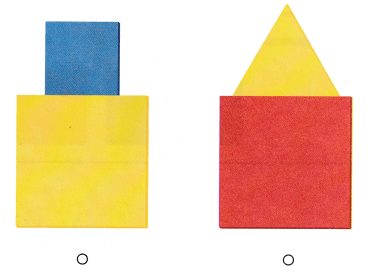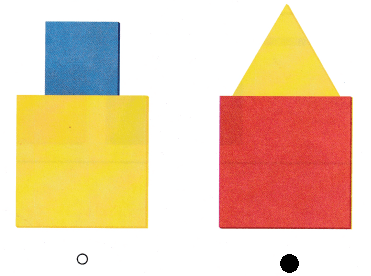# Texas Go Math Kindergarten Lesson 17.5 Answer Key Create Shapes

Refer to our Texas Go Math Kindergarten Answer Key Pdf to score good marks in the exams. Test yourself by practicing the problems from Texas Go Math Kindergarten Lesson 17.5 Answer Key Create Shapes.

## Texas Go Math Kindergarten Lesson 17.5 Answer Key Create Shapes

Essential Question
can you solve problems using the strategy draw a picture?

Unlock the ProblemExplanation:
A rectangle and a triangle are joined
to make the given shape.

Directions
Which shapes can be joined to make the larger shape? Circle the shapes. Trace and color to show the shapes that you used.

Try Another Problem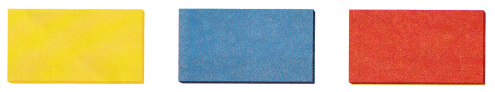Question 1.Explanation:
Two rectangles are joined one on the other
to make a square
square has all equal sides.

Question 2.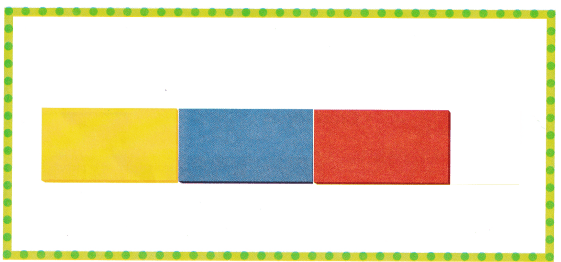Explanation:
Three rectangles are added side by side to make a larger rectangle
Opposite sides are equal in a rectangle.

Directions
1. How con you join some of the small rectangles to make a square? Use the rectangle shapes to make a square. Draw the square you make. 2. How can you join some rectangles to make a large rectangle that is not a square? Use the rectangle shapes to make a large rectangle. Draw the rectangle you make.

Share and Show

Question 3.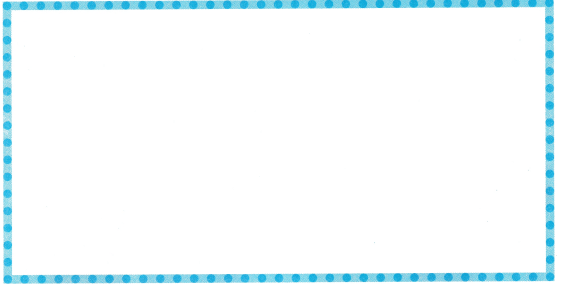Explanation:
In the above crown red colored as a rectangle
triangles in blue and circles in yellow.

Question 4.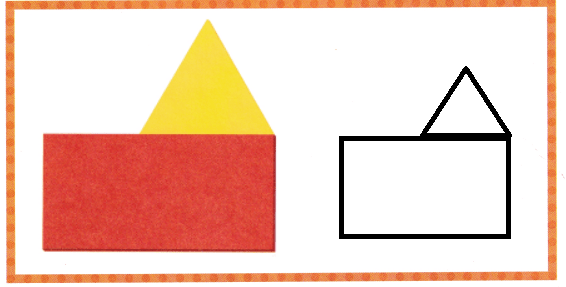Explanation:
A rectangle and triangle are used to make a house shape
down we used a rectangle on top we used a triangle.

Directions
3. Use rectangles and triangles from the two-dimensional shapes to make a crown, Draw the crown you make. 4. Use 3 different two-dimensional shapes. Join them together to make a new shape. Trace and draw the shape you make.

Question 5.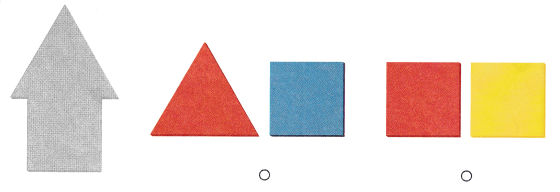Explanation:
The gray shape looks like a arrow
The combination of square and a triangle makes a arrow.

Question 6.Explanation:
In the first group there are two squares
In the second group there is a square and a triangle
so, marked the first group.

Question 7.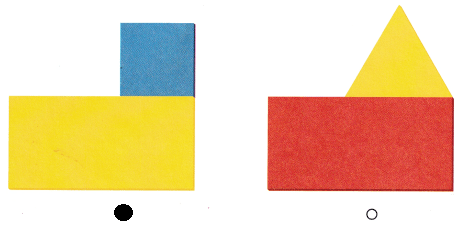Explanation:
In first group there is a square and a rectangle
in second group there is a triangle and a rectangle
so, marked the first group.

Directions
Choose the correct answer. 5. Which two shapes were used to make the gray shape? 6. Jackson uses two shapes to make a rectangle. Which two shapes does he use? 7. Which shows a shape mode by joining two rectangles?

### Texas Go Math Kindergarten Lesson 17.5 Homework and Practice

Question 1.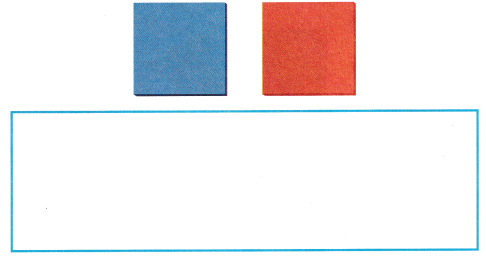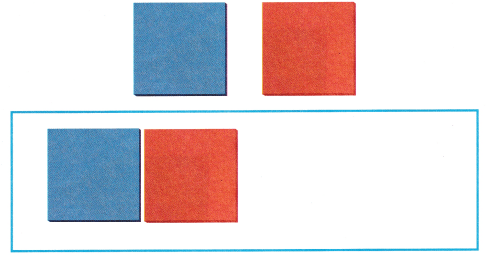Explanation:
Two squares are joined to form a rectangle
the squares are joined side by side

Question 2.Explanation:
Three rectangles are added side by side to make a larger rectangle
Opposite sides are equal in a rectangle.

Directions
1. How can you join the two squares to make a rectangle? Draw the rectangle. 2. How can you join some rectangles to make a larger rectangle? Draw the rectangle you make.

Texas Test Prep

Question 3.Explanation:
The gray shape looks like a arrow
The combination of square and a triangle makes a arrow.
so, marked the first group.

Question 4.